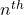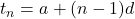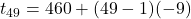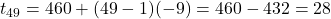## The first 3 terms of a sequence are given. Round to the nearest thousandth (if necessary) 460,451,442 find the 49th term

Question

The first 3 terms of a sequence are given. Round to the nearest thousandth (if necessary) 460,451,442 find the 49th term

in progress 0
5 months 2021-08-09T16:37:23+00:00 1 Answers 17 views 0

The  49th term of the given sequence

t₄₉ = 28

Step-by-step explanation:

Step(i):

Given sequence  460, 451,442,….

first term  a = 460

The difference of two terms of given sequence

d = 451 -460 = -9

d   = 442 -451 = -9

and so on

The given sequence is in arthematic progression(A.P)

Step(ii):-

Theof the given sequencen = 49 , a = 460 and d = -9

The  49th term